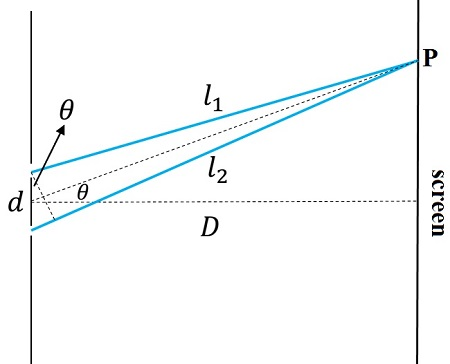# Light from a sodium lamp (wavelength = 598) illuminates two narrow slits. The fringe spacing on a...

## Question:

Light from a sodium lamp (wavelength = 598) illuminates two narrow slits. The fringe spacing on a screen 150 cm behind the slits is 4.0 mm. What is the spacing (in mm) between the two slits? Express your answer using two significant figures.

## Coherence and interference experiments

To obtain an interference pattern from two light sources is necessary that the sources have spatial and temporal coherence. For incoherent sources the phase difference between the waves behaves erratically and when the intensity due to the superposition of the waves is averaged over time results in a mean value not corresponding to the interference maxima or minima.

Temporal coherence is achieved if the sources have the same frequency. On the other hand, the spatial coherence is achieved if the sources are held at rest and so is the screen where the interference pattern is obtained. The usual way to guarantee the coherence of the sources is to split a single wavefront into two different fronts acting like new emitters of electromagnetic waves. Examples of interference devices based on this principle are Lloyd's mirror, Fresnel's prism, and Young's double-slit experiment. The first is based on the law of reflection, the second on the refraction and the third one in splits the wavefront by making it pass through the narrow slits (a diffractive mechanism).

The setup for Young's experiment is depicted in the figure below.The light emmited by waves emmited by the two point sources are gathered at the screen and the interference effects studied. Interference maxima are achieved if the optical path difference between the two waves, {eq}\Delta l {/eq}, is proportional to an integer number of wavelenghts,

with {eq}m=0,\pm 1,\pm 2, \dots {/eq}.

The optical path difference toward a screen whose distance, {eq}D {/eq}, is much larger than the separation between the slits, {eq}d {/eq},

is,

where {eq}\theta {/eq} describes the angular position of the maxima.

Consequently, joining the expressions (1) and (3) the resulting equation for the interference maxima is,

{eq}d\sin\theta=m\lambda {/eq}.

For small angles, like the ones resulting under condition (2) the sine of the angle can be replaced by the angle measured in radians,

{eq}d\theta=m\lambda {/eq}.

The position of the maxima is measured reative to a origin placed on the screen in such way that the position,

{eq}y_0=0 \; \text{correspoonds to} \; \theta=0 {/eq}.

The maxima are labeled {eq}y_{\pm 1},\;y_{\pm 2},\;y_{\pm 3}, \dots {/eq} in terms of their linear position. The maxima position in terms of the angle is computed as,

{eq}y_{i}=D\tan\theta_i {/eq},

since the tangent of the angular position equals the ratio of the linear position and the distance to the screen (see diagram).

The distance between consecutive maxima is,

{eq}\delta y=y_{i+1}-y_i=D(\tan\theta_{i+1}-\tan\theta_{i}) {/eq}.

Finally, for small angles the tangent is equivalent to the sine and equivalent to the angle measured in radians,

{eq}\tan\theta\sim\sin\theta\sim\theta {/eq},

hence,

{eq}\delta y=D(\theta_{i+1}-\theta_{i}) {/eq},

substituting from equation (4) for the orders {eq}m=i {/eq} and {eq}m=i+1 {/eq},

{eq}\delta y=D((m+1)\dfrac{\lambda}{d}-m\dfrac{\lambda}{d})=D\dfrac{\lambda}{d} {/eq}.

Solving for the distance between the slits {eq}d {/eq},

{eq}d=\dfrac{D\lambda}{\Delta y} {/eq}.

With the fringe spacing {eq}\Delta y=4 {/eq} mm, the distance to the screen {eq}D=150 {/eq} cm and the wavelength {eq}\lambda=598 {/eq} nm, the separation between the slits is,

{eq}d=2.2\times 10^{-4} {/eq} m or {eq}d=0.22 {/eq} mm.